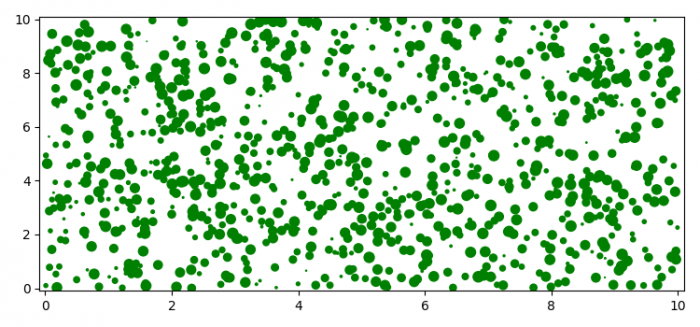# How to plot thousands of circles quickly in Matplotlib?

To plot thousands of circles quickly in Matplotlib, we will have to use matplotlib.collections. In this case, we will use CircleCollection.

## Steps

• Import the collections package from matplotlib along with pyplot and numpy.
• Set the figure size and adjust the padding between and around the subplots.
• Initialize variables "num" for number of small circles and "sizes" for sizes of circles.
• Create a list of circle patches.
• Add circle patch artist on the current axis.
• Set the margins of the axes.
• To display the figure, use show() method.

## Example

import numpy as np
import matplotlib.pyplot as plt
import matplotlib.collections as mc

plt.rcParams["figure.figsize"] = [7.50, 3.50]
plt.rcParams["figure.autolayout"] = True

num = 1000
sizes = 50 * np.random.random(num)
xy = 10 * np.random.random((num, 2))

patches = [plt.Circle(center, size) for center, size in zip(xy, sizes)]

fig, ax = plt.subplots()

collection = mc.CircleCollection(sizes, offsets=xy, transOffset=ax.transData, color='green')
plt.show()